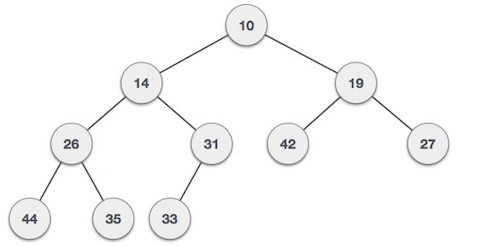# Heap Data Structures

Heap is a special case of balanced binary tree data structure where the root-node key is compared with its children and arranged accordingly. If α has child node β then −

key(α) ≥ key(β)

As the value of parent is greater than that of child, this property generates Max Heap. Based on this criteria, a heap can be of two types −

```For Input → 35 33 42 10 14 19 27 44 26 31
```

Min-Heap − Where the value of the root node is less than or equal to either of its children.Max-Heap − Where the value of the root node is greater than or equal to either of its children.Both trees are constructed using the same input and order of arrival.

## Max Heap Construction Algorithm

We shall use the same example to demonstrate how a Max Heap is created. The procedure to create Min Heap is similar but we go for min values instead of max values.

We are going to derive an algorithm for max heap by inserting one element at a time. At any point of time, heap must maintain its property. While insertion, we also assume that we are inserting a node in an already heapified tree.

```Step 1 − Create a new node at the end of heap.
Step 2 − Assign new value to the node.
Step 3 − Compare the value of this child node with its parent.
Step 4 − If value of parent is less than child, then swap them.
Step 5 − Repeat step 3 & 4 until Heap property holds.
```

Note − In Min Heap construction algorithm, we expect the value of the parent node to be less than that of the child node.

Let's understand Max Heap construction by an animated illustration. We consider the same input sample that we used earlier.## Max Heap Deletion Algorithm

Let us derive an algorithm to delete from max heap. Deletion in Max (or Min) Heap always happens at the root to remove the Maximum (or minimum) value.

```Step 1 − Remove root node.
Step 2 − Move the last element of last level to root.
Step 3 − Compare the value of this child node with its parent.
Step 4 − If value of parent is less than child, then swap them.
Step 5 − Repeat step 3 & 4 until Heap property holds.
```